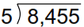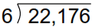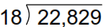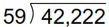# Grade 5 Multiplication & Division Worksheets

## Multiply & divide with grade 5 worksheets

These grade 5 worksheets provide more challenging practice on multiplication and division concepts learned in earlier grades.

## Multiplication

Multiply by 10, 100 or 1,000 with missing factors ____ x 98 =98,000
Distributive property 8 x 22 = 8 x 20 + 8 x 2 =
Multiply in parts 5 x 71 =
Multiply numbers near 100 9 x 104 =
Multiply 1-digit by 3-digit numbers 8 x 223 =

## Division

Divide 3 and 4 digit numbers by 1-digit mentally 812 ÷ 4 =
Division with remainder, within 1-100 68 ÷ 12 =
Division with remainder, within 1-1,000 981 ÷ 8 =
Division with remainder, divisor a whole ten 99 ÷ 30 =
Division with remainder, divisor a whole hundred 5,652 ÷ 700 =

## Multiplication in columns

Multiply 4-digit x 1-digit 4,481
x 2

Multiply 3-digit x 2-digit 457
x 21

Multiply 4-digit x 2-digit 2,345
x 23

Multiply 3-digit x 3-digit 456
x 789

## Long Division

Long division (no remainder)Long division with remainderLong division with remainders (divisors 10-25)Long division with remainders (divisors 10-99)Missing dividend or divisor problems 1,564 ÷ ___ = 34
Missing factor problems (solve by long division) ___ x 35 = 1,680

## Word problems

Mixed 4 operations word problems Word problems